# Test: Network Theorems - 2

## 10 Questions MCQ Test Network Theory (Electric Circuits) | Test: Network Theorems - 2

Description
Attempt Test: Network Theorems - 2 | 10 questions in 30 minutes | Mock test for Electrical Engineering (EE) preparation | Free important questions MCQ to study Network Theory (Electric Circuits) for Electrical Engineering (EE) Exam | Download free PDF with solutions
QUESTION: 1

### i1 = ?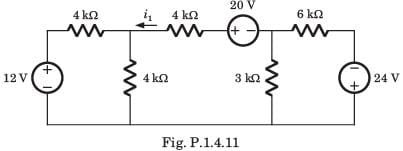Solution:

If we solve this circuit direct, we have to deal with three variable. But by simple manipulation variable can be reduced to one. By changing the LHS and RHS in Thevenin equivalent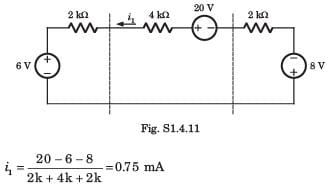QUESTION: 2

### A circuit is given in fig. P.1.4.12–13. Find the Thevenin equivalent as given in question..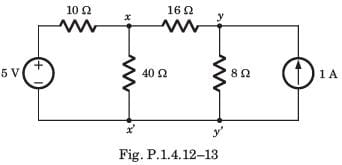Q. As viewed from terminal x and x' is

Solution:

We Thevenized the left side of xx' and source transformed right side of yy'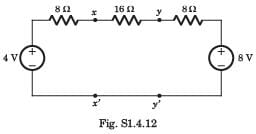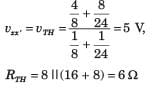QUESTION: 3

### A circuit is given in fig. P.1.4.12–13. Find the Thevenin equivalent as given in question..As viewed from terminal y and y' is

Solution:

Thevenin equivalent seen from terminal yy' is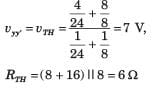QUESTION: 4

A practical DC current source provide 20 kW to a 50 Ω load and 20 kW to a 200 Ω load. The maximum power, that can drawn from it, is

Solution: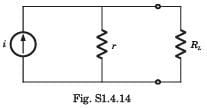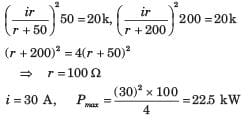QUESTION: 5

In the circuit of fig. P.1.4.15–16 when R = 0 Ω , the current iR equals 10 A.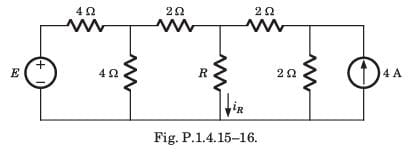Q. The value of R, for which it absorbs maximum power, is

Solution:

Thevenized the circuit across R, RTH = 2 Ω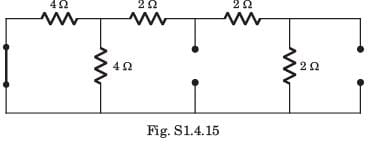QUESTION: 6

In the circuit of fig. P.1.4.15–16 when R = 0 Ω , the current iR equals 10 A.Q. The maximum power will be

Solution: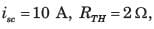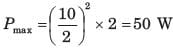QUESTION: 7

If vs1 = 6 V and vs 2 = -6 V then the value of vα is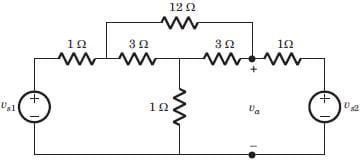Solution:

Since both source have opposite polarity, hence short circuit the all straight-through connection as shown in fig. S.1.4.33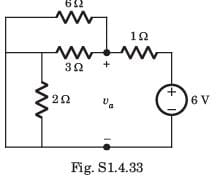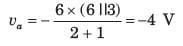QUESTION: 8

A network N feeds a resistance R as shown in fig.P1.4.34. Let the power consumed by R be P.If an identical network is added as shown in figure, the power consumed by R will be​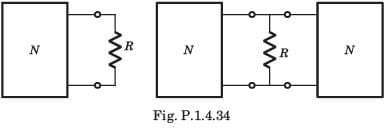Solution:

Let Thevenin equivalent of both network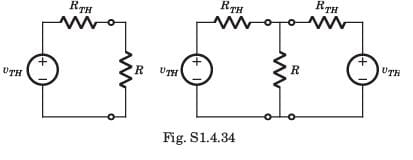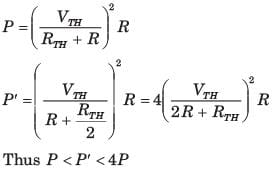QUESTION: 9

A certain network consists of a large number of ideal linear resistors, one of which is R and two constant ideal source. The power consumed by R is P1 when only the first source is active, and P2 when only the second source is active. If both sources are active simultaneously, then the power consumed by R is

Solution: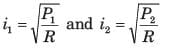using superposition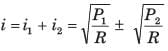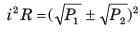QUESTION: 10

A battery has a short-circuit current of 30 A and an open circuit voltage of 24 V. If the battery is connected to an electric bulb of resistance 2 Ω, the power dissipated by the bulb is

Solution: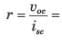0.8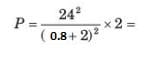146.93Use Code STAYHOME200 and get INR 200 additional OFF Use Coupon Code